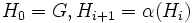Difference between revisions of "Artinian implies co-Hopfian"

This article gives the statement and possibly, proof, of an implication relation between two group properties. That is, it states that every group satisfying the first group property (i.e., Artinian group) must also satisfy the second group property (i.e., co-Hopfian group)
View all group property implications | View all group property non-implications
Get more facts about Artinian group|Get more facts about co-Hopfian group

Statement

Any Artinian group (i.e., a group satisfying the descending chain condition on subgroups) is co-Hopfian: it is not isomorphic to any proper subgroup of itself.

Definitions used

Artinian group

Further information: Artinian group

A group$G$ is termed Artinian if it satisfies the following equivalent conditions:

• If$H_0 \ge H_1 \ge H_2 \ge \dots \ge H_n \ge \dots$ is a descending chain of subgroups, there is a$n$ such that$H_n = H_m$ for all$m \ge n$.
• Any nonempty collection of subgroups of$G$ has a minimal element: a subgroup not containing any other member of that collection.

co-Hopfian group

Further information: co-Hopfian group

A group$G$ is termed co-Hopfian if there is no proper subgroup of$G$ isomorphic to$G$.

Proof

We prove the contrapositive here: if a group is not co-Hopfian, it is not Artinian.

Given: A group$G$ that is not co-Hopfian.

To prove:$G$ is not Artinian.

Proof: Suppose$H \le G$ is a subgroup and$\alpha:G \to H$ is an isomorphism (such a subgroup exists because$G$ is not co-Hopfian). Define:$H_0 = G, H_{i+1} = \alpha(H_i)$.

We prove by induction that$H_{i+1}$ is a proper subgroup of$H_i$ for each$i$. The base case is direct, since$H_1 = \alpha(H_0) = H < H_0 = G$.

For the induction, suppose$H_i < H_{i-1}$. Since$\alpha$ is an isomorphism, it preserves strictness of inclusions, and we thus have:$\alpha(H_i) < \alpha(H_{i-1}) \qquad \implies H_{i+1} < H_i$.

Thus, we have a strictly descending chain of subgroups of$G$ that does not stabilize at any finite stage. Thus,$G$ is not Artinian.